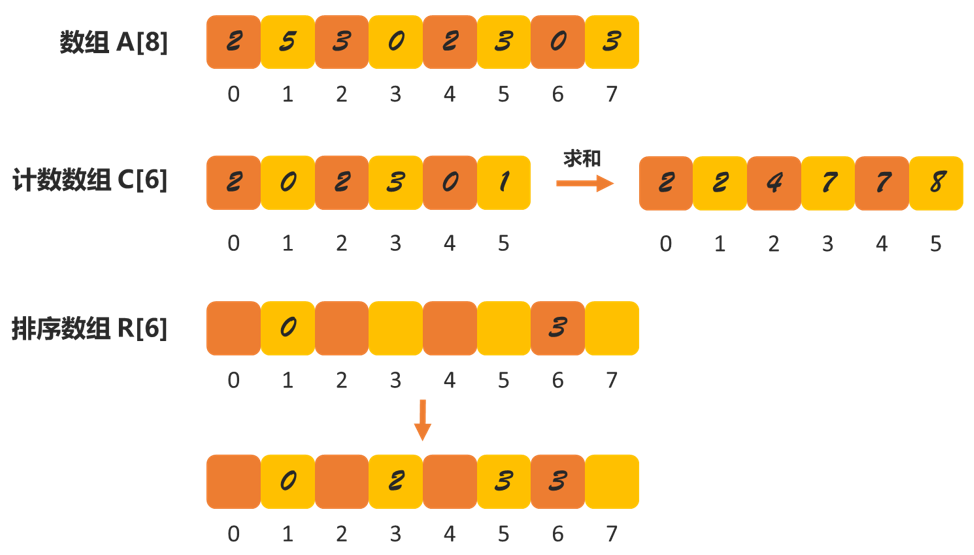# 1. 桶排序（Bucket sort）

$n$ 个数据分到 $m$ 个桶内，每个桶会有 $k=n/m$ 个元素，每个桶内使用快速排序。桶排序的时间复杂度为 $O(mklogk)$，如果桶的数量越接近 $n$，就会退变成 $O(n)$

1. 桶与桶之间有天然大小顺序；
2. 数据在桶之间的分布均匀。

# 2. 计数排序（Counting sort）

1. 如果数据范围是 [-1000, 1000]，那么我们给每一个数据加 1000，范围就变为 [0, 2000]；
2. 如果数据精度是小数点后一位如，[0.1, 9.8]，我们可以给每个数据乘以 10，就变为 [1, 98]。1. 因为数据的范围在 [0, 5]，所以把数据分为 6 个桶，并存放在 C 数组中（C 的下标代表数据，对应位置存放的是下标对应数据的数量）；
2. 然后对 C 中每个元素都求其前面元素与当前元素的和，即 C[i] = C[i - 1] + C[i]；
3. 请求一个存放排序好的数据的数组，与 A 同样大小；
4. 从右往左对 A 排序，重复一下步骤：
• R[C[A[n]] - 1] = A[n]
• n = n - 1

# copyright (c) strongnine

def countingSort(a):
n = len(a)
if n <= 1:
return

max = a
for i in range(1, n):
if max < a[i]:
max = a[i]

c = [0 for i in range(max + 1)]

for i in range(n):
c[a[i]] += 1

for i in range(1, max + 1):
c[i] = c[i - 1] + c[i]

r = [0 for i in range(n)]

for i in range(n - 1, -1, -1):
index = c[a[i]] - 1
r[index] = a[i]
c[a[i]] -= 1

return r

if __name__ == '__main__':
a = [2, 5, 3, 0, 2, 3, 0, 3]
r = countingSort(a)
print(r)



1. 数据的「位」需要可以独立分割出来比较；
2. 位之间有递进关系，即高位比低位高，就可以忽略更低位的大小。

# 4. 线性排序的局限

03-276608

#### 数据结构

04-253764

#### 数据结构总结06-114412

#### 数据结构——线性结构总结

04-149649

#### 数据结构的常用八种排序算法

05-022804

#### 数据结构之排序：排序基本概念和各种排序方法总结

11-301539

#### 数据结构之线性结构（向量与列表）

05-02768

#### 数据结构——图（1）很详细

09-086766

#### 数据结构与算法—拓扑排序

10-24173

#### 数据结构---线性排序（规律数据，线性复杂度）

11-264447

#### 数据结构（非线性表）©️2020 CSDN 皮肤主题: 技术黑板 设计师: CSDN官方博客点击重新获取扫码支付1.余额是钱包充值的虚拟货币，按照1:1的比例进行支付金额的抵扣。
2.余额无法直接购买下载，可以购买VIP、C币套餐、付费专栏及课程。余额充值